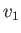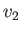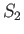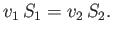Next: Bernoulli's Theorem Up: Incompressible Inviscid Flow Previous: Introduction

# Streamlines, Stream Tubes, and Stream Filaments

A line drawn in a fluid such that its tangent at each point is parallel to the local fluid velocity is called a streamline. The aggregate of all the streamlines at a given instance in time constitutes the instantaneous flow pattern. The streamlines drawn through each point of a closed curve constitute a stream tube. Finally, a stream filament is defined as a stream tube whose cross-section is a curve of infinitesimal dimensions.

If the flow is unsteady then the configuration of the stream tubes and filaments changes from time to time. However, if the flow is steady then the stream tubes and filaments are stationary. In the latter case, a stream tube acts like an actual tube through which the fluid is flowing. This follows because there can be no flow across the walls, and into the tube, because the flow is, by definition, always tangential to these walls. Moreover, the walls are fixed in space and time, because the motion is steady. Thus, the motion of the fluid within the tube would be unchanged were the walls replaced by a rigid frictionless boundary.

Consider a stream filament of an incompressible fluid whose motion is steady. Suppose that the cross-sectional area of the filament is sufficiently small that the fluid velocity is the same at each point on the cross-section. Moreover, let the cross-section be everywhere normal to the direction of this common velocity. Suppose thatandare the flow speeds at two points on the filament where the cross-sectional areas areand, respectively. Consider the section of the filament lying between these points. Because the fluid is incompressible, the same volume of fluid must flow into one end of the section, in a given time interval, as flows out of the other, which implies that(4.1)

This is the simplest manifestation of the equation of fluid continuity discussed in Section 1.9. The previous result is equivalent to the statement that the product of the flow speed and cross-sectional area is constant along any stream filament of an incompressible fluid in steady motion. Thus, a stream filament within such a fluid cannot terminate unless the speed at that point becomes infinite. Leaving this case out of consideration, it follows that stream filaments in steadily flowing incompressible fluids either form closed loops, or terminate at the boundaries of the fluid. The same is, of course, true of streamlines.Next: Bernoulli's Theorem Up: Incompressible Inviscid Flow Previous: Introduction
Richard Fitzpatrick 2016-03-31